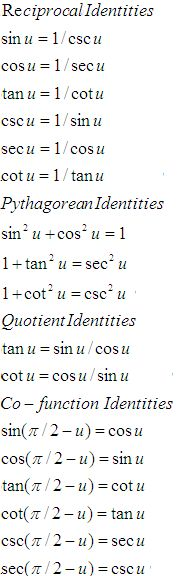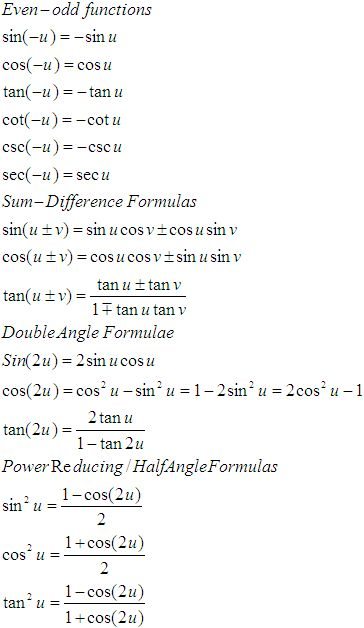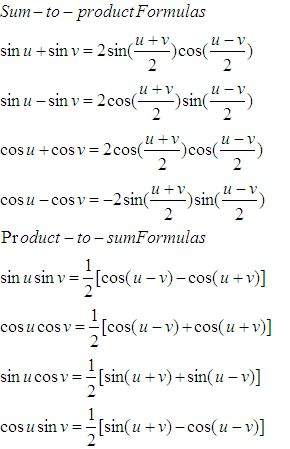Email us to get an instant 20% discount on highly effective K-12 Math & English kwizNET Programs!

Online Quiz (WorksheetABCD)

Questions Per Quiz = 2 4 6 8 10

High School Mathematics - 210.18 Trignometry Review

 Function 0o 30� 45� 60� 90� sin �0/2 �1/2 �2/2 �3/2 �4/2 cos �4/2 �3/2 �2/2 �1/2 0 tan 0 �3/3 1 �3 undefined sec 1 2�3/3 �2 2 undefined csc undefined 2 �2 2�3/3 1 cot undefined �3 1 �3/3 0

 sin cos tan 0 0 1 0 90 1 0 infinity 180 0 -1 0 270 -1 0 infinityFunctions of angles in all quadrants in terms of those in quadrant 1
 -A 900 � A P/2 � A 1800 � A P � A 2700 � A 3P/2 � A 3600 � A 2P � A sin -sinA cos A �sin A -cosA �sin A cos cos A �sin A -cos A �sin A cos A tan -tan A �cot A � tan A �cot A �tan A csc -csc A sec A � csc A -sec A �csc A sec sec A � csc A -sec A �csc A sec A cot -cot A � tan A � cot A �tan A � cot A

 Q 1: What is the angle of elevation of the sun when the length of the shadow of a pole is 31/2 times the height of the pole?60o30o90o Q 2: The angle of elevation of the top of a tower which is yet incomplete at a point 120 metres from its base is 45o. How much higher should it be raised so that the elevation at the same point may become 60o?87.84 metres100 metres36.85 metres Q 3: In triangle ABC b = 4, c = 6, B = 30o, find sin C.4/33/41/2 Q 4: The angles of a triangle ABC are in AP and b:c = 31/2:21/2. Find angle A.45 degrees60 degrees75 degrees Q 5: From the light house the angles of depression of two ships on opposite sides of the light house are observed to be 30o and 45o. If the height of the light house is 300 metres, find the distance between the ships if the line joining them passes through foot of the light house.819.6 metres809 metres800 metres Q 6: If tan C = 11, find sin C. cos C.Answer: Q 7: Find the degree measure of 3/4.45o42057l18ll35o Q 8: The sines of the angles of a triangle are in the ratio of 4:5:6, find the ratio of the cosines of the angles.4:5:212:9:22:3:4 Question 9: This question is available to subscribers only! Question 10: This question is available to subscribers only!